#### 期刊菜单

Analysis of Factors Affecting Fuel Efficiency
DOI: 10.12677/CCE.2018.61001, PDF, HTML, XML, 下载: 879  浏览: 3,118

Abstract: With the rapid development of the automotive industry, energy and environmental issues have fol-lowed, and it is of great significance to improve the fuel efficiency of automotive engines. This article is based on vehicle displacement, horsepower, vehicle length, vehicle weight and other indicators, using fuel efficiency as a dependent variable, and vehicle index as an independent variable to establish a regression model. Focusing on the problem of multicollinearity in the model, we try to use the variable selection method, stepwise regression method, principal component regression method, Ridge regression and Lasso, and partial least squares method to improve the common multiple linear regression model, and compare various methods. The advantages and disadvantages are selected from the best methods.

1. 引言

2. 符号说明及数据预处理

2.1. 符号说明

2.2. 数据预处理

3. 多元线性回归模型Table 2. Description of missing values (NA indicates missing values)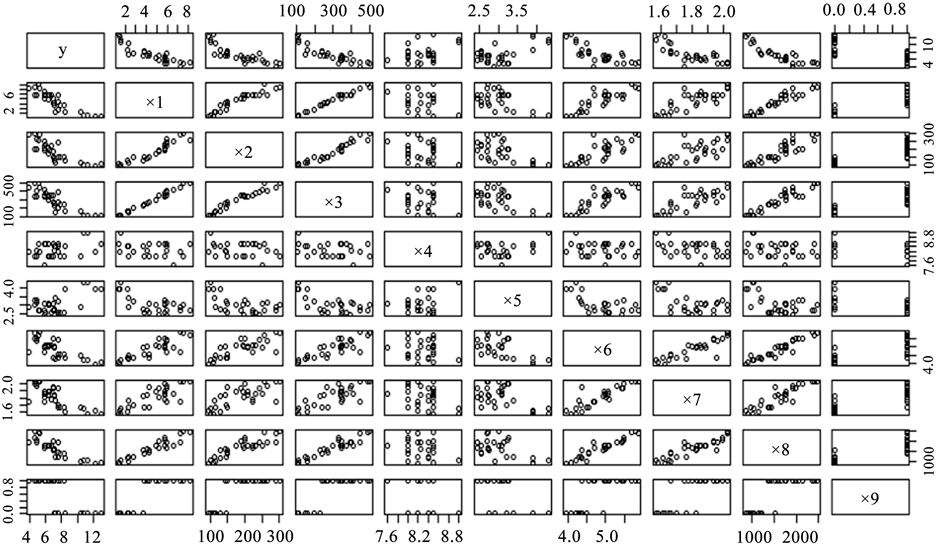Figure 1. Scatterplot matrix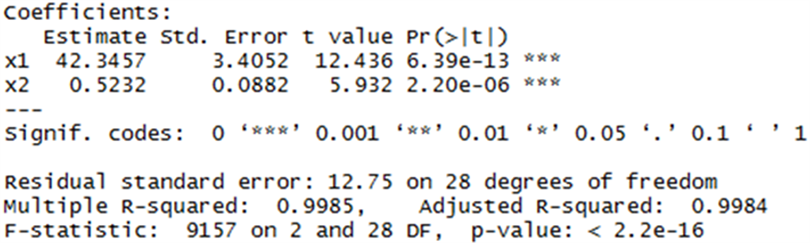Figure 2. Regression results (x3 is dependent variable)Table 3. Correlation coefficient matrix

${x}_{1}、{x}_{2}、{x}_{3}、{x}_{6}、{x}_{7}、{x}_{8}$ 具有很明显的负相关关系。y与 ${x}_{4}、{x}_{5}$ 具有正相关关系。 ${x}_{9}$ 为定性变量，所以并没有计算。因此可以尝试拟合全模型。

4. 多重共线性问题的解决

4.1. 所有子集法

${x}_{6}$ 的回归系数符号仍然与实际相反， ${x}_{6}、{x}_{8}$ 的方差膨胀因子依然很大(分别为9.96和9.87)，变量选择后并没有消除多重共线性(见图5)。

4.2. 逐步回归法Figure 3. Regression results (y is dependent variable)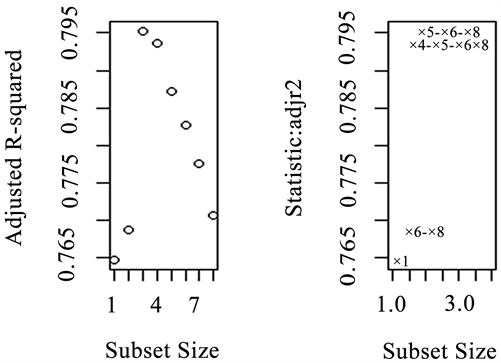Figure 4. Plots of adj R2 against subset size for the best subset of each sizeTable 4. Variance inflation factor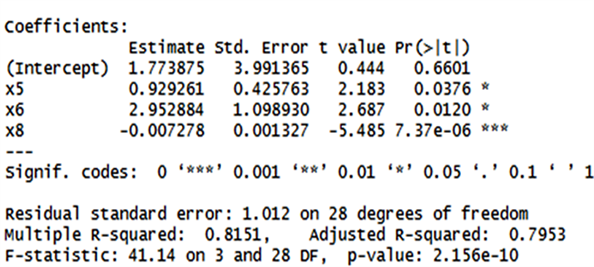Figure 5. Regression results of all possible subsets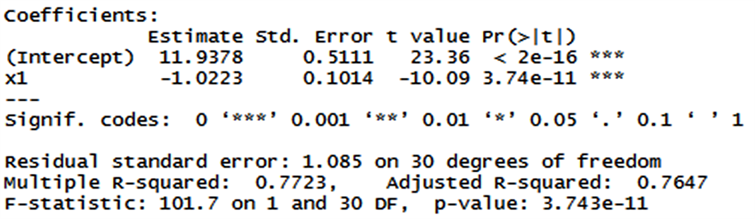Figure 6. Regression results of stepwise methods

4.3. 岭回归和lasso

4.3.1 . 岭回归

$\text{sy}=-0.3549s{x}_{1}-0.0564s{x}_{2}-0.3197s{x}_{3}+0.0613s{x}_{4}+0.1040s{x}_{5}-0.1334s{x}_{7}-0.2422s{x}_{8}$

4.3.2 . Lasso

Lasso算法改进了最小二乘法，在估计回归系数的同时可以达到变量选择的目的  。是受约束的最小二乘法，考虑P个自变量的回归模型，在 ${\sum }_{j=1}^{p}|{\beta }_{j}|\le s,\text{\hspace{0.17em}}\left(s\ge 0\right)$ 的约束条件下，使得残差平方和最小，

$\mathrm{min}{\sum }_{i=1}^{n}{\left\{{y}_{i}-\left({\beta }_{0}+{\beta }_{1}{x}_{1i}+\cdots +{\beta }_{p}{x}_{pi}\right)\right\}}^{2}$

$\mathrm{min}{\sum }_{i-1}^{n}{\left\{{y}_{i}-\left({\beta }_{0}+{\beta }_{1}{x}_{1i}+\cdots +{\beta }_{p}{x}_{pi}\right)\right\}}^{2}\text{+}\lambda {\sum }_{j=1}^{p}|{\beta }_{j}|,\text{\hspace{0.17em}}\lambda \ge 0$

K折交叉验证是评价模型的一种常用方法，它把所有的观测数据大致分为k等份，然后轮流以其中的所有可能的k−1份为训练集，用来拟合数据，剩下的一份为测试集，一共计算k次，得到拟合测试集时的均方误差那样的k个指标再做平均，对于每个模型都做一遍，然后选择平均均方误差最小的模型  。根据交叉验证，最佳 $\lambda$ 的简约模型是选择了 ${x}_{1}$ 和x8 (见图8)。

$\text{sy}=-0.5206s{x}_{1}-0.2287s{x}_{8}$

Lasso做参数估计的同时也起到了了变量选择作用，选出的简约模型保留了 ${x}_{1}$${x}_{8}$ ，符号也是正确的。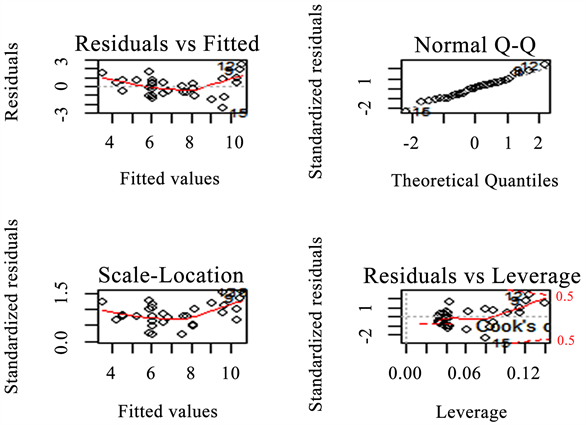Figure 7. Model diagnostic plots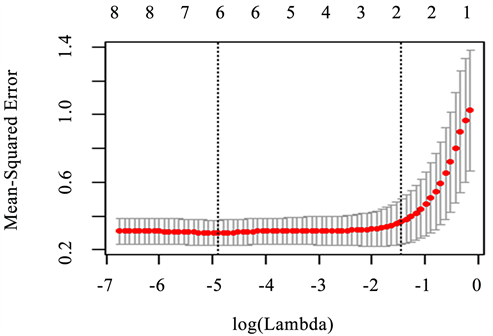Figure 8. Cross validation

4.4. 主成分回归法

4.4.1 . 主成分回归

$\stackrel{^}{\gamma }=V\stackrel{^}{\beta }$ 可求回归结果为：

$\text{sy}=-0.1545s{x}_{1}-0.1481s{x}_{2}-0.1549s{x}_{3}+0.0616s{x}_{4}+0.1056s{x}_{5}-0.1465s{x}_{6}-0.0842s{x}_{7}-0.1526s{x}_{8}$

4.4.2 . 不完全主成分回归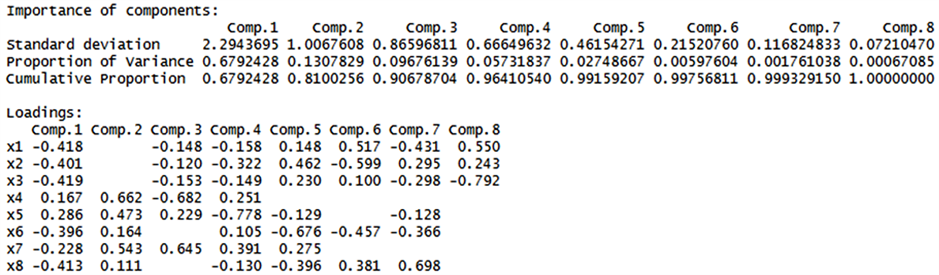Figure 9. Principal component analysis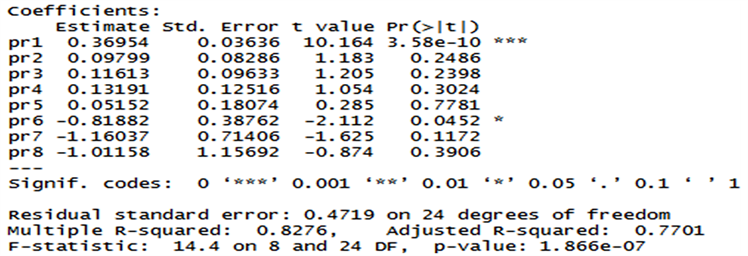Figure 10. Principal component regression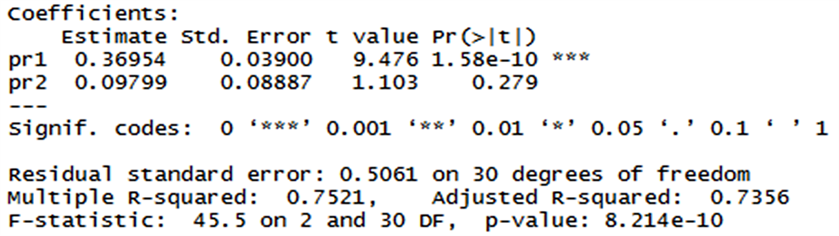Figure 11. Incomplete principal component regression

$\text{sy}=-0.1565s{x}_{1}-0.1428s{x}_{2}-0.1554s{x}_{3}+0.1264s{x}_{4}+0.1519s{x}_{5}-0.1304s{x}_{6}-0.0310s{x}_{7}-0.1417s{x}_{8}$

4.5. 偏最小二乘回归

$\text{sy}=-0.1625s{x}_{1}-0.1492s{x}_{2}-0.1585s{x}_{3}+0.0660s{x}_{4}+0.11s{x}_{5}-0.1396s{x}_{6}-0.0594s{x}_{7}-0.1596s{x}_{8}$

4.6. 比较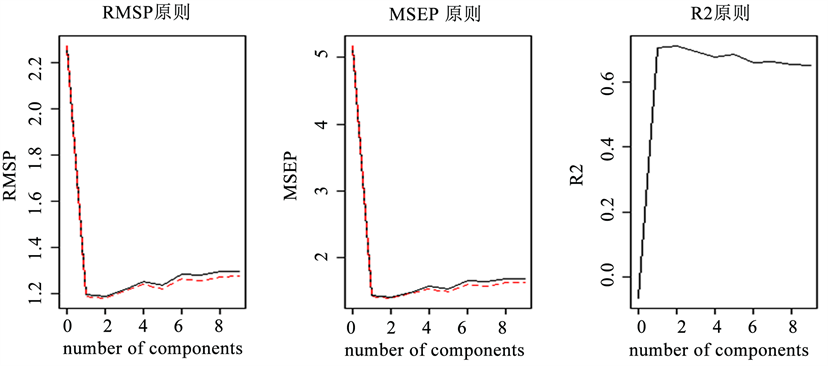Figure 12. Partial least squares regression cross validation results

5. 结论

NOTES

*通讯作者。

  潘虹如. 燃油税改革: 小排量、新能源汽车受宠[J]. 标准生活, 2009(1): 41-43.  王登峰, 邓阳庆, 刘延林, 等. 汽车使用诸因素对燃油经济性的影响分析与试验研究[J]. 公路交通科技, 2008, 25(8): 150-153.  陈海涛. 汽车结构因素对燃油经济性的影响[J]. 公路与汽运, 2006(2): 1-4.  陈晓停, 曹兰杰, 汪金花. 岭估计在多项式曲面拟合GNSS高程中的应用[J]. 河北联合大学学报(自然科学版), 2016, 38(4): 1-6.  张秀秀, 王慧, 田双双, 等. 高维数据回归分析中基于LASSO的自变量选择[J]. 中国卫生统计, 2013, 30(6): 922-926.  龙泽海, 杨毅, 赵月丽. 基于lasso方法的银行对中小企业贷款供给意愿研究[J]. 金融与经济, 2017(3): 58-65.  Massy, W.F. (1965) Principal Components Regression in Exploratory Statistical Research. Publications of the American Statistical Association, 60, 234-256. https://doi.org/10.1080/01621459.1965.10480787  林育贤, 冯圣红. 基于PCA法的BP网络对冰蓄冷系统的空调负荷预测[J]. 建筑节能, 2016(7): 13-15.  龙泽海, 杨毅, 赵月丽. 基于lasso方法的银行对中小企业贷款供给意愿研究[J]. 金融与经济, 2017(3): 58-65.